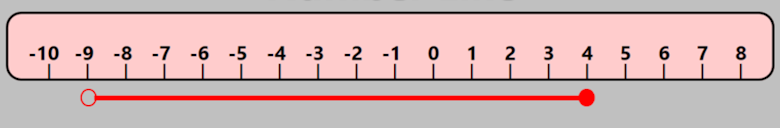# Exam-Style Questions.

## Problems adapted from questions set for previous Mathematics exams.

### 1.

GCSE Higher

The red line in the diagram below shows an inequality. If the variable is $$x$$, which inequality best describes $$x$$?(a) $$-9 \le x \le 4$$

(b) $$-9 \lt x \le 4$$

(c) $$-9 \le x \lt 4$$

(d) $$-9 \lt x \lt 4$$

(e) $$-4 \le x \le 9$$

### 2.

GCSE Higher

(a) Solve 9m < 15m − 12

(b) On the number line below, show the set of values of $$x$$ for which $$-4 \le x-1 \lt 5$$### 3.

GCSE Higher

Solve the following inequalities then explain how the whole number solutions to A and B different.

$$A: 5 \le 5x \lt 30$$ $$B: 5 \lt 5x \le 30$$

### 4.

GCSE Higher

John times how long it takes him to run around Hazelnut Park each Friday afternoon. The last three weeks his times, rounded to the nearest minute are $$p, q \; \text{and} \; r$$.

He notices that $$q$$ is 5% more than $$p$$ and $$r$$ is 5% less than $$q$$.

Indicate which of the following statements are true:

(a) $$r \lt p$$ and $$r \lt q$$

(b) $$r \gt p$$ and $$r \gt q$$

(c) $$r = p$$ and $$r \lt q$$

(d) $$p \gt q$$ and $$p = r$$

(e) $$p =q$$ and $$p \gt r$$

If you would like space on the right of the question to write out the solution try this Thinning Feature. It will collapse the text into the left half of your screen but large diagrams will remain unchanged.

The exam-style questions appearing on this site are based on those set in previous examinations (or sample assessment papers for future examinations) by the major examination boards. The wording, diagrams and figures used in these questions have been changed from the originals so that students can have fresh, relevant problem solving practice even if they have previously worked through the related exam paper.

The solutions to the questions on this website are only available to those who have a Transum Subscription.

Exam-Style Questions Main Page

Search for exam-style questions containing a particular word or phrase:

To search the entire Transum website use the search box in the grey area below.